Obtain the data you need to make the most informed decisions by accessing our extensive portfolio of information, analytics, and expertise. Sign in to the product or service center of your choice.

# IPRs and unconventional reservoirs

How has the shale revolution affected analysis of reservoir performance?

The industry has changed dramatically since the advent of the shale revolution in North America, enabled by the application of horizontal drilling and multi-stage hydraulic fracturing to allow commercial production of hydrocarbon from ultra-low permeability reservoirs in resource plays.

In this post, IHS will discuss how this situation affected the way we analyze, evaluate, characterize and quantify the reservoir performance as people make decisions to sustain and optimize hydrocarbon production.

Let's start by discussing how we analyze conventional wells (wells completed in high permeability reservoirs--for example, sandstones). In these cases, the permeability facilitates the fluid flow across the formation. So as the pressure in the wellbore reduces, the reservoir fluids in the vicinity will move with a flow behavior which is characterized by different flow regimes, determined by the different stages in production:

• Transient flow: This represents the early stage of production where the pressure propagates from the well into the formation without any restrictions (behaving as an "infinite-acting" system with no boundaries). Since at this stage the complete reservoir volume has not yet been contacted, the external pressure will stay constant as the drainage area continuously grows. Figure 1 below shows this scenario.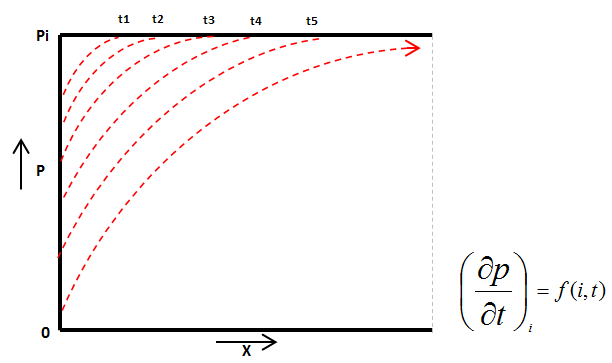Figure 1: Pressure propagation in transient flow

Assuming we have a vertical well (with radial flow geometry), we can solve the diffusivity equation to obtain an expression of rate vs time: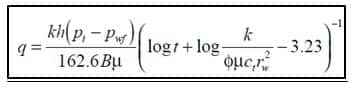Equation 1: Transient flow solution for a vertical well

• Boundary-dominated flow (BDF): After the pressure propagation reaches the boundaries of the system, the drainage area is equal to the full reservoir volume. In this condition there are alternative scenarios illustrated in Figures 2 and 3 which show the evolution of the reservoir pressure profile vs space.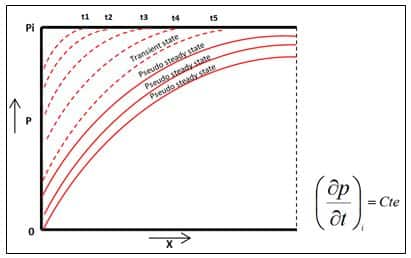Figure 2: Pseudo-steady state (constant rate)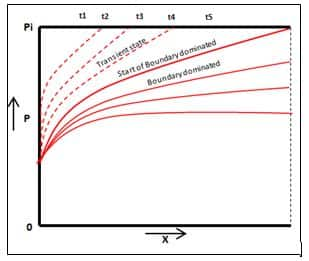Figure 3: Boundary dominated flow (constant pressure

If the external pressure in the boundary declines uniformly at a constant production rate, this period is known as pseudo-steadystate, and it is typical of a closed system (see Figure 2).

Alternatively, the static pressure could be declining at the boundary but not uniformly, because the flow rate is not constant. This is also often called "tank-type flow".

The expression of boundary-dominated flow describes an equivalent situation where the pressure propagation has contacted the whole reservoir volume but in this case we are producing at a constant bottom-hole pressure (see Figure 3).

Assuming we have a vertical well (with radial flow geometry), we can solve the diffusivity equation for a constant flowing pressure to obtain a rate solution. This equation is particularly useful as it allows obtaining a direct relationship between the rate and the average reservoir pressure: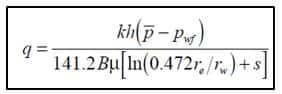Equation 2: Boundary-dominated flow solution for a vertical well

There is another scenario where after the whole reservoir volume is contacted, the static pressure does not decline but stays constant, generating a stable pressure profile; we refer to this flow regime as steady-state. This is the typical behavior of wells with a pressure support, for example from a strong aquifer influx or waterflooding (see Figure 4).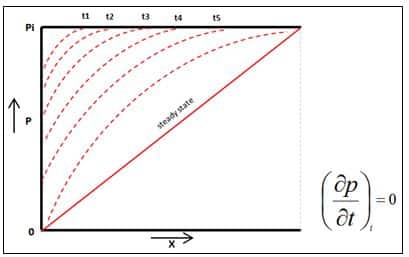Assuming a vertical well with radial flow geometry, we can obtain a rate expression through this equation: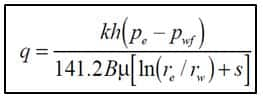Equation 3: Steady-state flow solution for a vertical well

It is worth noting that for most conventional systems (high permeability), the transient flow condition lasts a relatively short period of time (typically a few days or weeks) until the pressure reaches the boundaries, where a fully defined drainage area is contacted and the classical flow solution for boundary-dominated flow can be applied.

In contrast, in unconventional reservoirs the transient flow regime can last for several months or even years; by applying Equation 1 it can be observed that with a lower permeability, it takes more time for pressure to propagate in the reservoir; therefore, we would not be able to apply the classical solutions for these wells for most of the early life of their production.

Inflow Performance Relationship

The inflow performance relationship (IPR) defines a curve that relates the production flow rate with the reservoir energy. Basically, it defines the ability of the reservoir to provide volumes to the well at different flowing pressures, as shown in Figure 5.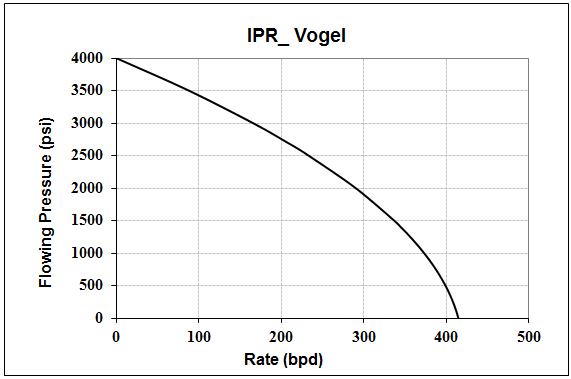Figure 5: IPR curve (Vogel correlation)

As the figure shows, for a given bottomhole flowing pressure (Pwf), the production rate can be obtained from the curve. However, the Pwf depends on the surface wellhead pressure (Pwh) which is controlled by operational decisions, for example choke management. Understanding the IPR curve is important to optimize the impact of operations and equipment on the well production and to quantify the real well deliverability.

The IPR will not be constant though; as the reservoir pressure declines, its energy depletes and hence will have a reduction in the reservoir inflow capacity, so any optimization using a given IPR curve will not be valid for the whole life of the well.

There are several IPR correlations developed by different authors and widely used in our industry (e.g., Darcy, Vogel, Fetkovich, Joshi, Economides, Rawlins and Schellhardt, Jones, among others). Although each correlation has distinctive assumptions (for example, well geometry and fluid type), most of them have been developed for pseudo-steady or steady state conditions (constant drainage area). They provide valuable information to quantify the maximum attainable production from a well at a given time to help us make decisions impacting the well operation, for example, artificial lift design.

In Figure 6, the Darcy model was applied in a well to represent the conditions of the production in which the average reservoir pressure is 3,700 psia (blue line) pointing at a maximum rate of 3,020 Bpd of liquid. A posterior analysis of the same well indicates a reduction of the maximum rate to 1,750 Bpd of liquid, given that the average reservoir pressure has decreased to 3,000 psia (red line).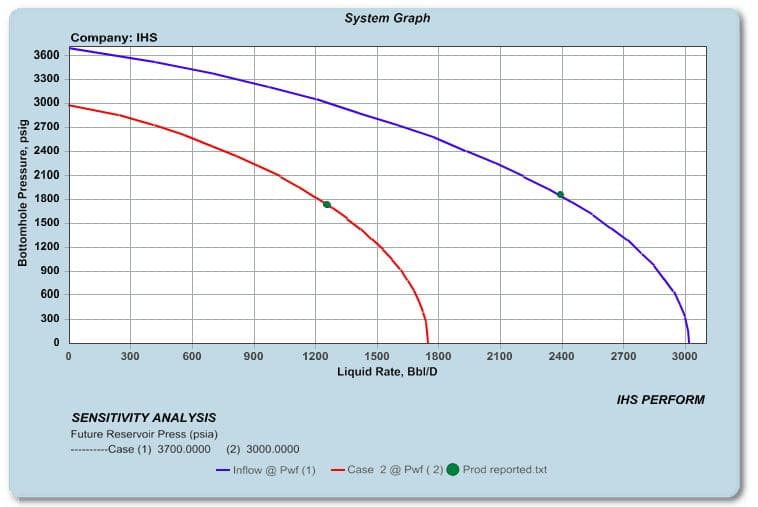Figure 6: IPRs with Average Reservoir Pressure depleted in time

Evaluating well performance in unconventional reservoirs

Unconventional reservoirs are typically produced with horizontal wells which are hydraulically fractured in multiple stages to improve the permeability in the well vicinity, as the original reservoir permeability is very low (nanoDarcy to microDarcy). This causes a Stimulated Reservoir Volume (SRV) which constitutes the true drive mechanism.

Figure 7 is a simplified representation of a well with these characteristics. The green area represents the original formation permeability, the yellow area represents the hydraulic fractures, and the white zone represents the stimulated zone with an improved permeability.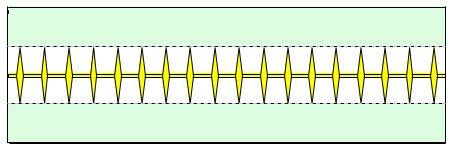Figure 7: Horizontal fractured well schematic

In the early life of the well, during transient flow, the flow geometry will be linear (as planar fractures dominate the pressure propagation, as Figure 8 illustrates) and the pressure drop will only occur in the SRV, not in the un-stimulated formation. This causes the response to behave as an infinite-acting system where the IPR behavior cannot be predicted with a simple equation.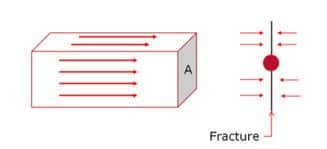Figure 8: Schematic of linear flow regime

In order to evaluate the reservoir influx, we can generate IPR curves based on the response of an analytical Rate Transient Analysis (RTA) model which captures all the relevant well and reservoir parameters but also honors the average pressure in the SRV (equivalent to the stabilized shut-in pressure if the well was hypothetically closed at that period of time); this better represents the transient nature of flow and allows a more realistic and reliable production optimization.

In Figure 9 we can see the actual bottomhole flowing pressure is 900 psia and the average reservoir pressure was estimated in 5,453 psia with a match obtained from the Enhanced Frac Region (EFR) model of an oil shale producing well.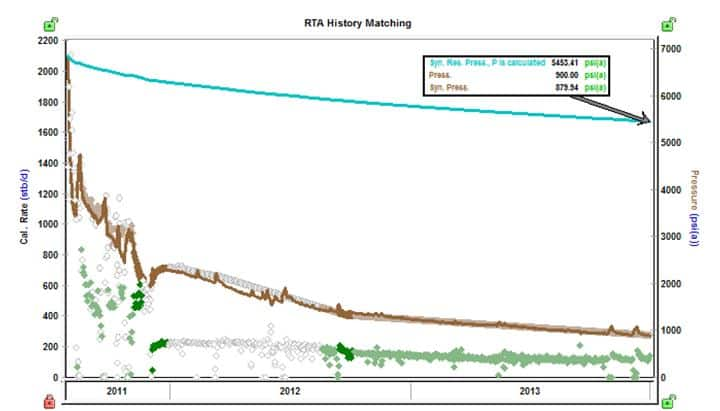Figure 9: Oil well History matched with an RTA model

Notably, if the well would be shut in for a month, then the model predicts that the pressure would not reach 5,453 psia but probably it would be closer to 1,300 psi. The resulting IPR is shown in Figure 10.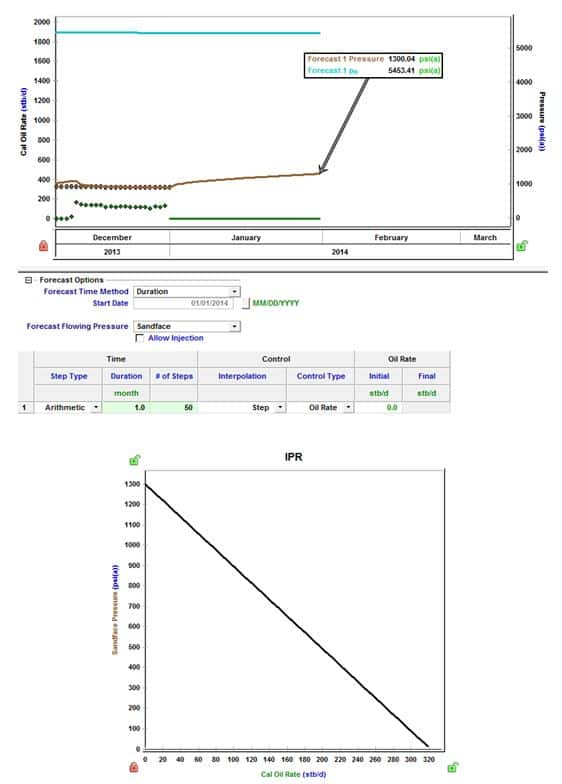Figure 10: IPR calculated from RTA model

In conclusion, this IPR can be used as a proper representation of the current well deliverability. It honors the transient flow regime, and it provides a very important link to connect the reservoir model with the production system. This provides benefits such as helping to optimize the size and timing of installation of tubing in the wellbore, or to correctly size pipeline and equipment (i.e compressors, pumps and any other artificial lift) for optimum well performance.